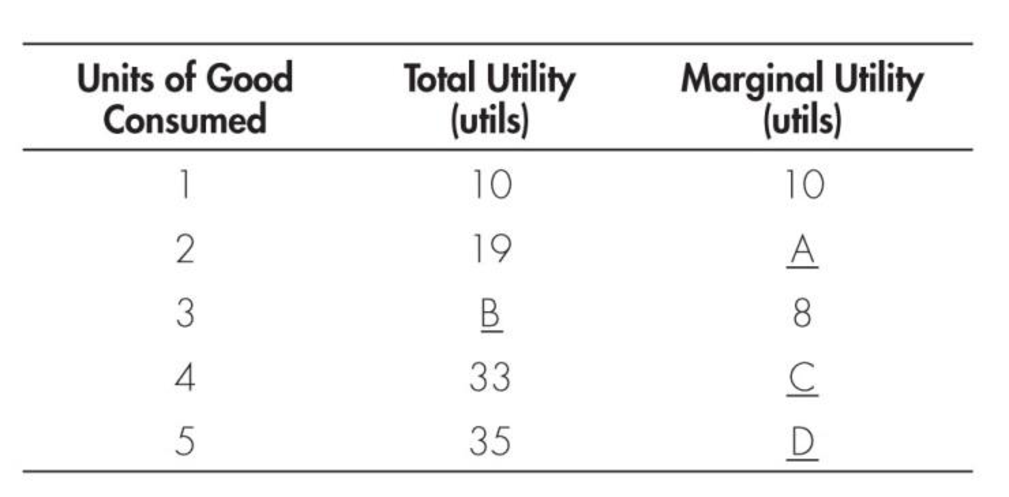Chapter 20, Problem 2WNG

Chapter
Section
Textbook Problem

Fill in blanks A–D in the following table.To determine

Identify the values of total utility and the marginal utility.

Explanation

Marginal utility (MU) can calculated using the following formula:

Marginal utility=Change in total utilityChange in quantity (1)

Substitute the respective values from the given table in Equation-1, to get the value of MU at Point A as follows:

Marginal utility=191021=91=9

Thus, MU at Point A is 9.

The value of total utility at Point B, or at 3 units can be calculated as follows:

Total utility3units=Total utility2units+Marginal utility3units=19+8=27

Thus, at Point B, the total utility is 27

Still sussing out bartleby?

Check out a sample textbook solution.

See a sample solution

The Solution to Your Study Problems

Bartleby provides explanations to thousands of textbook problems written by our experts, many with advanced degrees!

Get Started

Define the term marketing

MKTG 12:STUDENT ED.-TEXT

Explain the relationship between NPV and a firms value.

Managerial Accounting: The Cornerstone of Business Decision-Making

How do you compute the future value of an ordinary annuity?

Intermediate Accounting: Reporting And Analysis

EXPECTED INTEREST RATE The real risk-free rate is 3%. Inflation is expected to be 3% this year, 4% next year, a...

Fundamentals of Financial Management, Concise Edition (with Thomson ONE - Business School Edition, 1 term (6 months) Printed Access Card) (MindTap Course List)

What are the components of a typical business report?

Foundations of Business (MindTap Course List)